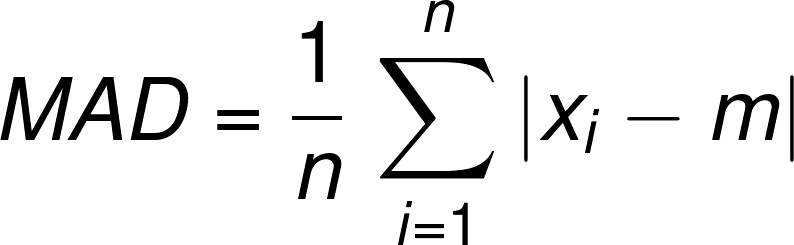# Mean Absolute Deviation Calculator

Created by Julia Żuławińska
Reviewed by Bogna Szyk and Jack Bowater
Last updated: Apr 06, 2022

The mean absolute deviation calculator is a tool that can help you quickly find the mean absolute deviation around the mean, median, or any other number. If you want to know what is mean absolute deviation - we break down the name in the article below. We also explain how to find it using a mean absolute deviation formula, or by following a few simple steps.

Now, input up to 50 numbers and get the mean absolute deviation for your dataset!

## What is mean absolute deviation? Mean absolute deviation calculator

The mean absolute deviation shows us how spread out the numbers are in a data set. But how? And what does deviation even mean?

• Deviation is the difference from what is usual.
• An absolute number takes the positive value of a number, without regards to its sign.
• Mean is an average of a set of numbers.

So, what is the mean absolute deviation? It's the average of every value's distance from a certain central point. This point can be a mean, median, mode, or any other statistically significant number.

To calculate it, use our mean absolute deviation calculator or do it on your own using the How to find mean absolute deviation? section below. How to use the MAD calculator? Select the central point. If you want it to be mean or median of your data set - the calculator will calculate it for you. If not, choose the option other and input an appropriate value. All that's left for you to do is input your data set and get your result!

## How to find mean absolute deviation (MAD)? Mean absolute deviation formula

If you are wondering how to find MAD, our calculator uses the mean absolute deviation formula:

MAD = Σ|xi - m| / n,

where:

• n is the amount of numbers in the set;
• xi is the ith number of the set; and
• m is a certain central point (mean, median, mode, etc)

So, to find mean absolute deviation, follow these steps:

1. Determine the central point: m.

2. Find the difference from the central point for each point: xi - m.

3. Calculate the absolute value of each difference: |xi - m|.

4. Find the mean of the absolute deviations - sum up all the absolute differences and divide them by the amount of data points: Σ|xi - m| / n.

Now, let's go to an example to see how to find the mean absolute deviation by using these steps.

## How to find MAD - an example

Let's calculate the mean absolute deviation for 6 friends' lost socks in the last year: 3, 17, 9, 7, 13, 11.

1. Let's say you want to calculate the MAD around the mean. In this case, the mean is the amount of socks lost, on average, by one person:

m = (3 + 17 + 9 + 7 + 13 + 11) / 6 = 60 / 6 = 10

1. Calculate the deviation from the mean:
• 3 - 10 = -7
• 17 - 10 = 7
• 9 - 10 = -1
• 7 - 10 - -3
• 13 - 10 = 3
• 11 - 10 = 1
1. Find the absolute value of each deviation:
• |-7| = 7
• |7| = 7
• |-1| = 1
• |-3| = 3
• |3| = 3
• |1| = 1
1. Find the mean of absolute values of each deviation:

MAD = (7 + 7 + 1 + 3 + 3 + 1) / 6 = 3.67

It means that, on average, the amount of lost socks differs from the mean by 3.67 "socks". What does it mean? It means that, in theory, each of 6 friends lost ten socks in the last year on average, while in reality this isn't the case - they lost anywhere between 6.33 and 13.67 socks (10±3.67).

Even if you now know how to find MAD by hand, you don't need to repeat these tedious calculation anymore. Just use our mean absolute deviation calculator!

• MAD can indicate mean absolute deviation or median absolute deviation. These are not the same. In the median absolute deviation, you calculate the median of the distances from a central point.
• The of data points can be also portrayed with standard deviation. Although similar in concept, standard deviation gives us the opportunity to further analyze a dataset with variance, estimate sample size, and so on.

Now you know what is mean absolute deviation and how to calculate it. Before you go, check out our other descriptive statistics like this percentile calculator!

Julia ŻuławińskaCentral point (m)
Mean
Data (Enter up to 50 numbers)
x₁
x₂
People also viewed…

### Christmas tree

Welcome to the Christmas tree calculator, where you will find how to decorate your Christmas tree in the best way. Take a look at the perfect Christmas tree formula prepared by math professors and improved by physicists. Plan in advance how many lights and decorations you'll need!

### Polynomial regression

The polynomial regression calculator can help you find a polynomial curve that best fits your data set.

### Sunbathing

Do you always remember to put on sunscreen before going outside? Are you sure that you use enough? The Sunbathing Calculator ☀ will tell you when's the time to go back under an umbrella not to suffer from a sunburn!

### Wilcoxon rank-sum test

The Wilcoxon rank-sum test calculator allows you to perform the famous non-parametric test based on the ranks of observations. Both exact and approximated versions are available, along with corrections for ties and continuity!